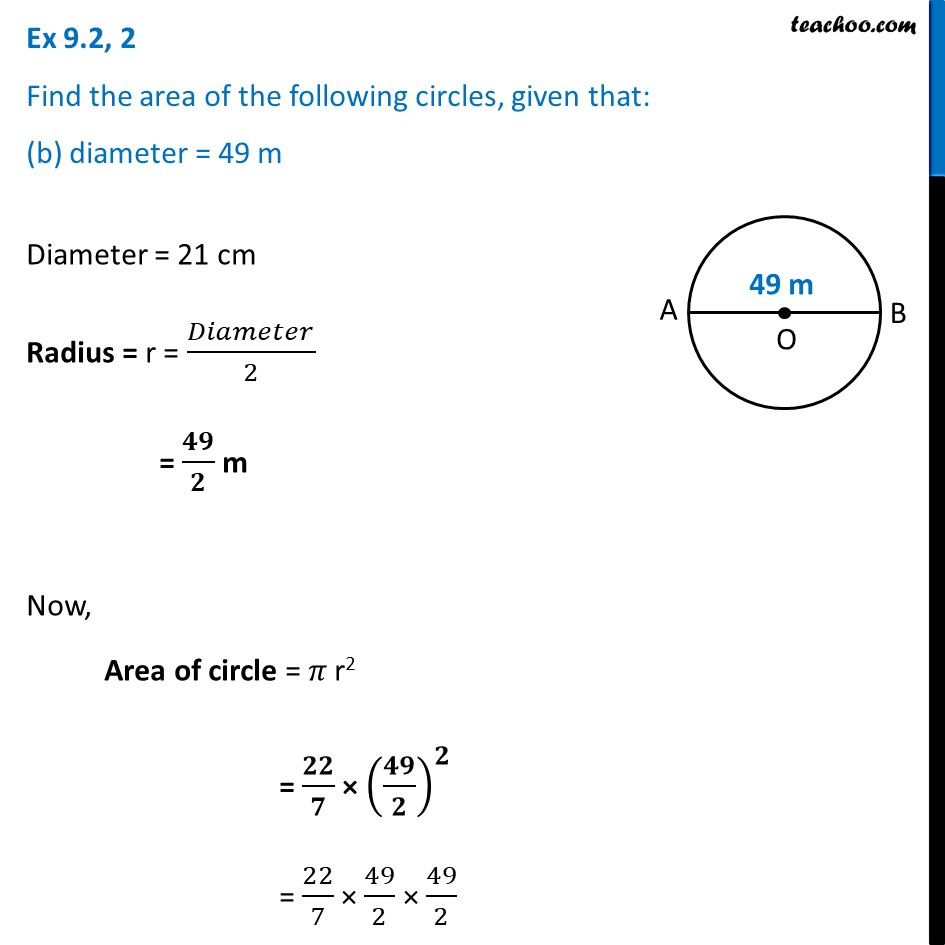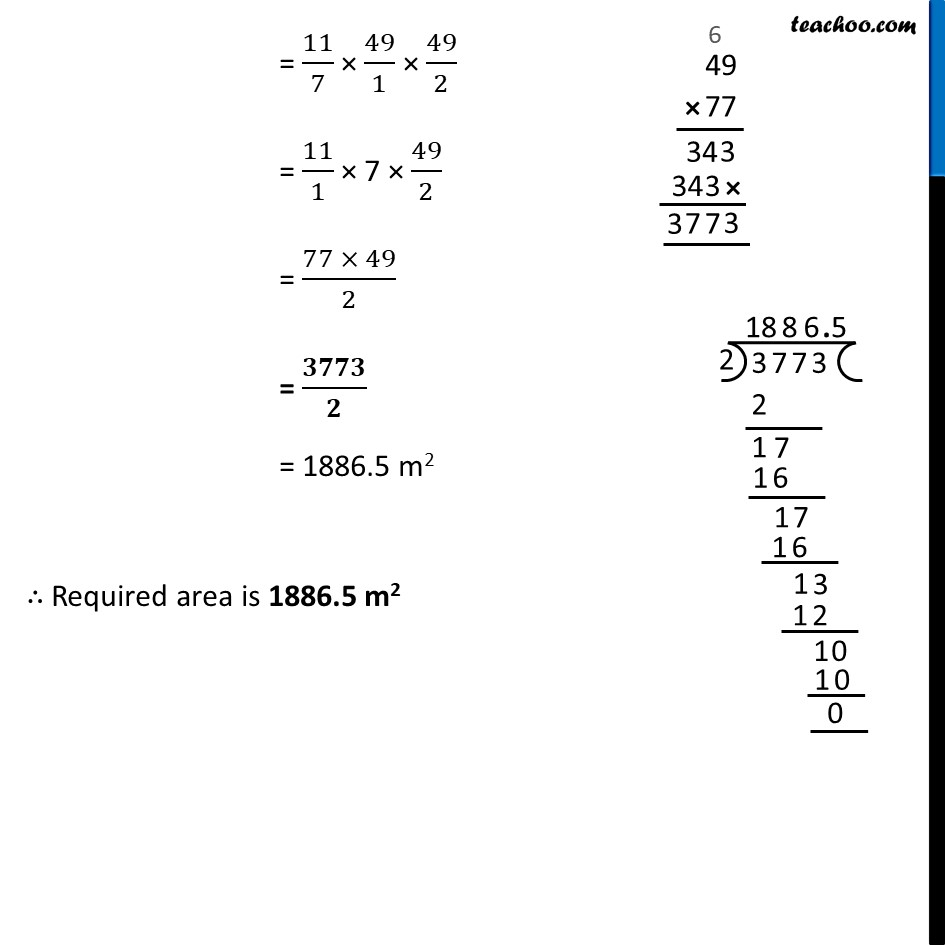Ex 9.2

Chapter 9 Class 7 Perimeter and Area
Serial order wiseLearn in your speed, with individual attention - Teachoo Maths 1-on-1 Class

### Transcript

Ex 9.2, 2 Find the area of the following circles, given that: (b) diameter = 49 mDiameter = 21 cm Radius = r = 𝐷𝑖𝑎𝑚𝑒𝑡𝑒𝑟/2 = 𝟒𝟗/𝟐 m Now, Area of circle = 𝜋 r2 = 𝟐𝟐/𝟕 × (𝟒𝟗/𝟐)^𝟐 = 22/7 × 49/2 × 49/2 = 11/7 × 49/1 × 49/2 = 11/1 × 7 × 49/2 = (77 × 49)/2 = 𝟑𝟕𝟕𝟑/𝟐 = 1886.5 m2 ∴ Required area is 1886.5 m2